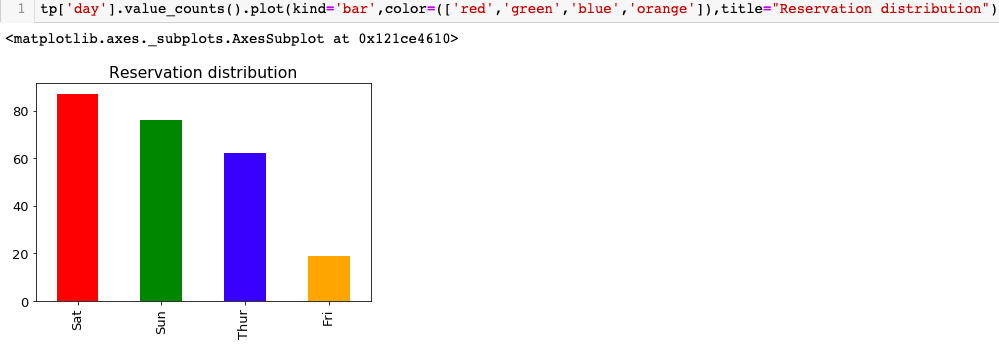# The art of Exploratory data analysis (EDA)

## What is Exploratory Data Analysis (EDA)?

As the name suggest, it is a technique to analyse the data by exploring it. EDA is one of the key as well as indispensable step in data analysis. This can be understood as a general checkup of patient (data) by the doctors (data enthusiast), before doing any surgery (analysis, modeling, prediction, classification, etc.).

## What are the goals of EDA?

EDA is done for two major underlying reasons, firstly to understand the data and secondly to identify faults or peculiar events (data points) in the dataset. Let’s try to understand what is meant by understanding and peculiar data points in detail.

To understand the data characteristics Understanding the data means answering following preliminary questions that generally pops up in the mind of data person on first encounter with a particular dataset:

• Where is this data coming from? (What population group or subject)
• What is the high level contained information? (e.g cost data, revenue, budget, employee salaries, customer data, usage data and so on.)
• What are the variables available?
• What does their value mean? (e.g average time per page would mean that out of all the time spent by user on the website in one session, how much time was spent on a every particular page)
• What population segment data belong to? (e.g The data of cancer patient would meant that out of all patients the collected data is of cancer patients only.
• What is the Sample distribution?
• What is the strength and direction of the relationships among input variable and outcome variable?
• What is the Central tendency of the data?
• What is the spread of the data?

— To identify discrepancies in the data → Discrepancies in data means

• Presence of incorrect values
• Missing values
• Outliers (probable outliers or possible outliers)
• Assumption violations

Based on the findings during EDA, a preliminary selection of the data can be done or the further course of actions can be decided to treat discrepancies.

# Part I — Categorical variables EDA

## How EDA is carried out?

As we have seen what and why of EDA, it time to answer how EDA is done? In this post we are going to focus on EDA techniques for categorical variables which includes ordinal, dichotomous and nominal variables. You can find details of types of variable here.

EDA can be graphical or quantitative depending on what are we trying to find. Following previous analogy of patient, reports like x-ray, MRI, scans, can be seen as graphical method which provides overall picture of data and involves qualitative analysis. Whereas blood reports, dimensions of tumour can be understood as quantitative method which are objective.

In general data is constitute of several columns and rows. Now one can choose to explore one variable at a time (uni+variate=univariate), two variables at a time (bi+variate=bivariate), or multiple variables at same time (multi+variate= multivariate).

# Univariate categorical EDA

## Univariate-Quantitative EDA:

The most useful information that can be extracted from this analysis in context to the categorical variable, is to know the categories, frequency of occurrence, proportion or percentage of data falls under each category. Frequency tables are the most popular way of doing this analysis.

## Univariate-Graphical EDA:

When we think graphs, bar plots are one of the most widely used graphs. A similar information from frequency tabulation can also be attained in form of bar plots, which are more useful in presenting the analysis. Having able to present the information in visual form is one of the key element in effectively presenting the analysis.Bar plot to show total number of reservation on particular weekday

# Multivariate Categorical EDA

## Multivariate-Quantitative EDA:

1. Cross tabulation: Cross-tabulation is the basic bivariate non-graphical EDA technique. However, it is not limited to the bivariate, but can be extended further. Reason why more than 5-way cross tables are not very popular is because it becomes a little hard to apprehend as the cross tabulation grows.

2. Univariate statistics per category: In case we have one categorical input variable and one quantitative outcome variable, generally the outcome variable statistics are calculated for each category and after that compare the statistics across the categories. The comparison is done using statistical tests such as anova.

## Multivariate-graphical EDA:

• Side-by-Side Box-plots: In case we want to explore categorical input variable and quantitative output variable, the approach is to separate all the cases based on categories and then make box plots of output variable. Side by side box-plots are useful in investigating the relationship between categorical and quantitative variable. In addition to it, distribution of outcome variable can also be seen at each categorical variable level.

EDA can bring enough understanding and knowledge to make aware decisions. However, one should not mistake EDA as an initial phase or one step process. As we progress with the data science cycle, one might need to do some EDA again after obtaining the results to analyse why the model is behaving in certain way or why a certain kind of output is seen. It wouldn’t be wrong to see EDA as process than as a phase.

Quantitative variables EDA

The complete code can be found on my Github. If you find this post helpful please let me know by comment section. You can also send me your suggestions and topics of your interest.

For Natural Language Processing enthusiasts, checkout EDA for NLP.

Never stop exploring!

--

--